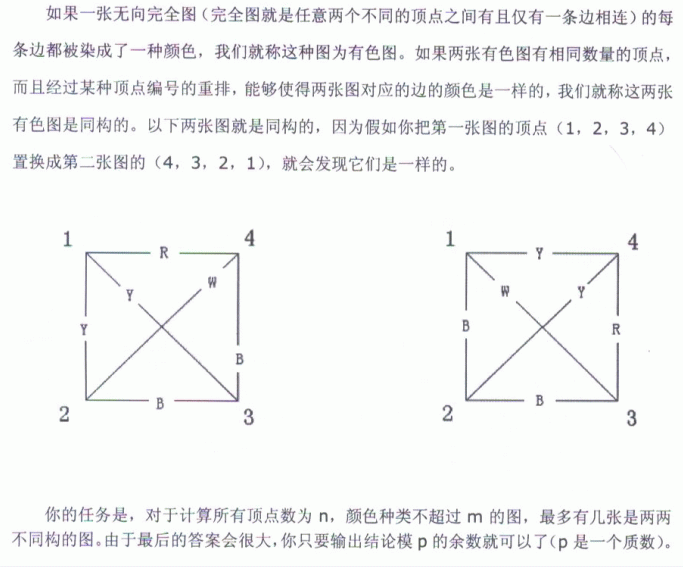DescriptionInput

输入三个整数N,M,P

1<=N<=53,1<=M<=1000,N< P<=10^ 9

即总数模P后的余数

Solutions

并不会如何把点循环转换成边循环，因此在看题解前只会多个$n$的做法qwq

这题有个比较显然的结论，就是一个点置换对应着一个唯一的边置换。而且，边置换是成群的。

基于这个结论，容易得到一个$n!$级别的算法，即暴力枚举点置换，并计算出对应的边置换，然后套用Polya定理解决。但显然，这种做法是TLE的。

由于Polya定理需要的只是循环数，而不是置换循环分解的形式。因此可以得到另外一个结论，对于同一个共轭类的置换，其对应的边置换循环数是一样的。

同时，共轭类的大小也易得到，其大小为$\frac{n!}{\prod{c_i(p) \cdot i^{c_i(p)}}}$；其中$c_i(p)$表示循环长度为$i$的循环的个数。

基于上诉两点，可以得到一个较为优秀的做法：枚举共轭类，并暴力求出边置换的循环数。时间复杂度是$O(kn^3)$，其中$k$表示共轭类的数量。通过暴力枚举可得知，当$n=53$时，$k$是5e5级别的。

看起来已经足够了，但还是TLE！而时间的瓶颈就在于求出边置换的循环数。

考虑优化这个过程。

对于一条边而言，有两种情况：一种为循环内的边，一种为两个循环之间的边。

若为循环内的边，其产生的循环数相当于节点数为$L$的完全图的置换，其个数为$\frac{L}{2}$

若为两个循环间的边，其产生的循环数相当于集合大小分别为$L_1,L_2$的完全二分图的置换，其个数为$gcd(L_1,L_2)$

时间复杂度$O(kn^2)$